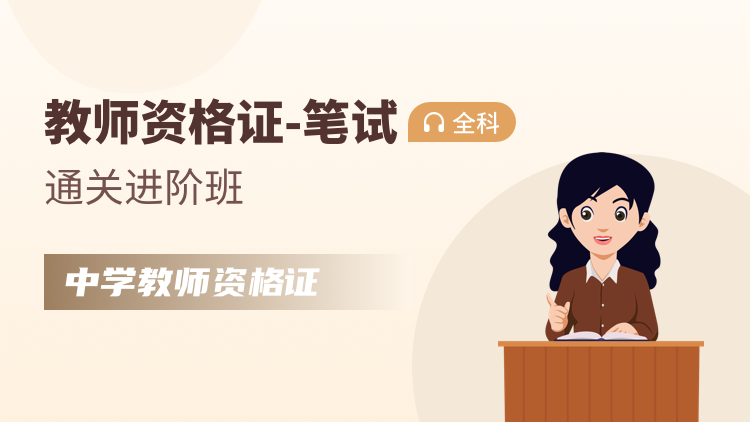¥800.00
365天
• 精讲
• 冲刺
• 真题
• 模拟
• 中学教育知识与能力
• 中学综合素质
• 课程介绍
• 课程表• 前言（一）
• 前言（二)
• 教育的产生与发展（一）
• 教育的产生与发展（二）
• 教育学的产生与发展（一）
• 教育学的产生与发展（二）
• 教育与人的发展（三）
• 教育与人的发展（四）
• 教育与社会的发展（一）
• 教育与社会的发展（二）
• 教育与人的发展（一）
• 教育与人的发展（二）
• 教育与人的发展（三）
• 教育与人的发展（四）
• 教育制度
• 教育目的（一）
• 教育目的（二）
• 教育目的（三）
• 教育科学研究（一）
• 教育科学研究（二）
• 中学课程（一）
• 中学课程（二）
• 中学课程（三）
• 中学课程（四）
• 中学课程（五）
• 中学课程（六）
• 中学课程（七）
• 中学课程（八）
• 中学教学（一）
• 中学教学（二）
• 中学教学（三）
• 中学教学（四）
• 中学教学（五）
• 中学教程（六）
• 中学教程（七）
• 中学教程（八）
• 中学教程（九）
• 认知过程（一）
• 认知过程（二）
• 认知过程（三）
• 认知过程（四）
• 认知过程（五）
• 认知过程（六）
• 认知过程（七）
• 认知过程（八）
• 认知过程（九）
• 认知过程（十）
• 认知过程（十一）
• 学习理论（一）
• 学习理论（二）
• 学习理论（三）
• 学习理论（四）
• 学习理论（五）
• 学习动机理论（一）
• 学习动机理论（二）
• 学习动机理论（三）
• 学习迁移（一）
• 学习迁移（二）
• 学习策略
• 知识学习与技能学习
• 中学生心理发展（一）
• 中学生心理发展（二）
• 中学生心理发展（三）
• 中学生心理发展（四）
• 中学生心理发展（五）
• 中学生心理发展（六）
• 中学生心理发展（七）
• 中学生心理发展（八）
• 中学生心理发展（九）
• 中学生心理发展（十）
• 心理辅导（一）
• 心理辅导（二）
• 心理辅导（三）
• 中学德育（一）
• 中学德育（二）
• 中学德育（三）
• 中学德育（四）
• 班级管理（一）
• 班级管理（二）
• 班级管理（三）
• 班级管理（四）
• 班级管理（五）
• 教师心理
• 模拟题（一）
• 模拟题（二）
• 模拟题（三）
• 模拟题（四）
• 模拟题（五）
• 模拟题（六）
• 模拟题（七）
• 模拟题（八）
• 模拟题（九）
• 模拟题（十）
• 第一节
• 第二节
• 第三节
• 第四节
• 第五节
• 第六节
• 第七节
• 18年真题一
• 18年真题二
• 18年真题三
• 18年真题四（完）
• 冲刺押题（一）
• 冲刺押题(二)
• 冲刺押题(三)
• 冲刺押题(四)
• 冲刺押题（五）
• 冲刺押题（六）
• 冲刺押题（七）
• 冲刺押题（八）
• 冲刺押题（九）

# 中学综合素质

###### 教材精讲试听 展开 收起
• 考情分析（一）
• 考情分析（二）
• 考情分析（三）
• 考情分析（四）
• 教育观（一）
• 教育观（二）
• 教育观（三）
• 教育观（四）
• 教育观（五）
• 教育观（六）
• 教育观（七）
• 学生观（一）
• 学生观（二）
• 学生观（三）
• 学生观（四）
• 教师观（一）
• 教师观（二）
• 教师观（三）
• 教师观（四）
• 教师观（五）
• 教育法律法规考情分析
• 教育法律法规概述（一）
• 教育法律法规概述（二）
• 教育法律法规概述（三）
• 教育法律法规概述（四）
• 师生的权利与义务（一）
• 师生的权利与义务（二）
• 重要教育法律法规条文（一）
• 重要教育法律法规条文（二）
• 重要教育法律法规条文（三）
• 重要教育法律法规条文（四）
• 重要教育法律法规条文（五）
• 重要教育法律法规条文（六）
• 重要教育法律法规条文（七）
• 重要教育法律法规条文（八）
• 重要教育法律法规条文（九）
• 重要教育法律法规条文（十）
• 重要教育法律法规条文（十一）
• 重要教育法律法规条文（十二）
• 重要教育法律法规条文（十三）
• 要教育法律法规条文（十四）
• 教师职业道德考情分析
• 教师职业道德规范（一）
• 教师职业道德规范（二）
• 教师职业道德规范（三）
• 职业道德规范（四）
• 教师职业道德规范（五）
• 教师职业道德规范（六）
• 教师职业道德规范（七）
• 教师职业行为（一）
• 教师职业行为（二）
• 文化素养考情分析
• 历史常识（一）
• 历史常识（二）
• 历史常识（三）
• 历史常识（四）
• 历史常识（五）
• 历史常识（六）
• 历史常识（七）
• 传统文化常识（一）
• 传统文化常识（二）
• 传统文化常识（三）
• 传统文化常识（四）
• 科学常识（一）
• 科学常识（二）
• 文学常识（一）
• 文学常识（二）
• 文学常识（三）
• 文学常识（四）
• 文学常识（五）
• 文艺常识
• 第五章 考情分析（一）
• 第五章 考情分析（二）
• 信息处理能力（一）
• 信息处理能力（二）
• 信息处理能力（三）
• 信息处理能力（四）
• 信息处理能力（五）
• 逻辑思维能力（一）
• 逻辑思维能力（二）
• 逻辑思维能力（三）
• 逻辑思维能力（四）
• 逻辑思维能力（五）
• 逻辑思维能力（六）
• 阅读理解能力（一）
• 阅读理解能力（二）
• 教育写作（一）
• 教育写作（二）
• 教育写作（三）
• 教育写作（四）
• 教育写作（五）
• 教育写作（六）
• 教育写作（七）
• 教育写作（八）
• 教育写作（九）
• 第一节
• 第二节
• 第三节
• 第四节
• 第五节
• 第六节
• 第七节
• 第八节
• 第九节
• 第十节
• 第十一节
• 第十二节
• 第十三节
• 第十四节
• 第十五节
• 第十六节
• 第十七节
• 第十八节
• 第一节
• 第二节
• 第三节
• 第四节
• 第五节
• 第六节
• 第七节
• 第八节
• 第九节
###### 冲刺预测 展开 收起
• 第一节
• 第二节
• 第三节
• 第四节
• 第五节
•昵称

时间

• 如何查看已购课程？

请用购课账号登录，即可查看课程；如提示签署协议，则点击立即签署，填写完毕提交成功后即可查看您的课程。

• 课程过期了怎么办？

在学习中心可点击订单查看有效期，过来有效期则无法观看； 如含有重学服务，可以在成绩公布之后第一时间内发送成绩单截图和用户名到重新开课。 如不含重学服务，可以拨打，联系网校工作人员二次购买，部分套餐网校会为您提供老学员专属优惠。